## Tuned Power Lines in Transmission:

Equation (5.23) characterizes the performance of a Tuned Power Lines in long Transmission line. For an overhead line shunt conductance G is always negligible and it is sufficiently accurate to neglect line resistance R as well. With this approximation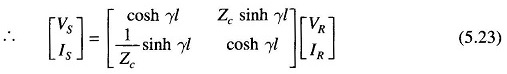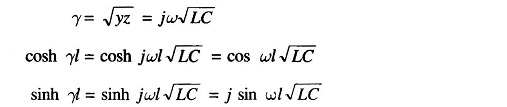Hence Eq. (5.23) simplifies to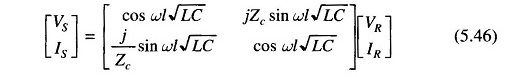Now if ωl√LC = nπ, n = 1, 2, 3, …i.e. the receiving-end voltage and current are numerically equal to the corresponding sending-end values, so that there is no voltage drop on load. Such a line is called a tuned line.
For 50 Hz, the length of line for tuning is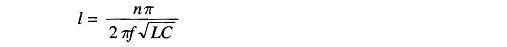Since 1/√LC ≈ v, the velocity of light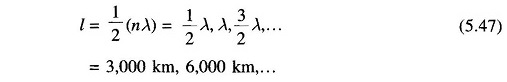It is too long a distance of transmission from the point of view of cost and efficiency (note that line resistance was neglected in the above analysis).

For a given line, length and frequency tuning can be achieved by increasing L or C, i.e. by adding series inductances or shunt capacitances at several places along the line length. The method is impractical and uneconomical for power frequency lines and is adopted for telephony where higher frequencies are employed.

A method of tuned power lines which is being presently experimented with, uses series capacitors to cancel the effect of the line inductance and shunt inductors to neutralize line capacitance.

A long line is divided into several sections which are individually tuned. However, so far the practical method of improving line regulation and power transfer capacity is to add series capacitors to reduce line inductance; shunt capacitors under heavy load conditions; and shunt inductors under light or no-load conditions.

Scroll to Top